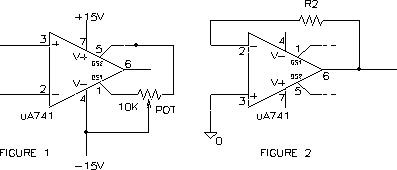EE 321 Lab

Lab 3: OPERATIONAL AMPLIFIERS PART II -- NON-IDEAL DC CHARACTERISTICS

Prelab for Lab 3

Do the prelab before coming to lab.

In this lab we will investigate some of the non-ideal DC characteristics of op amps. These are the effects of input offset voltage and bias currents, output voltage and current saturation. Throughout the experiment we will be comparing the 741 and 411 op amps.

Input Offset Voltage

1. Construct a single-stage 741 amplifier having an inverting gain of 1000 using a feedback resistor of 100 K ohm. Sketch the circuit. With the input grounded (zero input voltage), what is the d.c. level at the output? What `offset voltage' in series with the non-inverting input would account for the output level? How does this compare with the spec for the op amp?

2. Add a 10 K ohm pot as shown in Figure 1 and null the offset voltage to zero by adjusting the pot setting.3. Change either power supply voltage from by 1 Volt to determine the effect of the supply voltage changes on input offset voltage. How much does the input offset voltage change per volt of supply? Express this in dB. Compare this to the spec.

4. Remove the offset nulling circuitry. Briefly repeat part 1 for the 411 op amp. Are the two amps comparable with respect to input offset voltage?

Input Bias Current

5. Measure the bias current IB at the inverting input of the 741 op amp by inserting a large (10-30 M ohm) resistor in the feedback of a unity gain follower, as shown in Figure 2. Measure the output d.c. level. What bias current does this imply from the inverting input (magnitude and direction)? Compare with op amp specs.

6. Replace the 741 with a 411 op amp. What is the d.c. output voltage now? Both input bias current and input offset voltage contribute to this output voltage. How much contribution to this output offset voltage would you expect from your measured input offset voltage? How much of this offset voltage is due to the input bias current (find IB for the 411 in the spec sheet)?

Output Voltage and Current Limitations

7. Connect the 741 in a inverting amplifier with a gain of 10. At about 1 kHz frequency, vary the output amplitude into and out of saturation while observing the voltage at the (-) input. Why does the voltage at the inverting input suddenly depart from virtual ground? (Hint: Simultaneously display the (-) input and output waveforms.)

8. How much output current do you think your 741 op amp can deliver? 1 Amp? Investigate this question by applying a 100 ohm load resistance to the output of the op amp. Increase the output voltage until the waveform becomes noticeably affected. What peak output current does this imply? What does the spec sheet say about output current capability? Do the same for the 411 op amp. Which op amp delivers more current?

Comparison of op amps

9. From the data obtained so far, which type of op amp, the 741 or 411, is better, closer to ideal? Which has a more informative spec sheet?

Extra Credit

10. Op-amps do not like to have their (-) and (+) inputs shorted together. Try it for one of your op amps. What happens? Can you explain?